Next: SIMPLE ABNORMALITY THEORIES Up: APPLICATIONS OF CIRCUMSCRIPTION Previous: THE BLOCKS WORLD

# AN EXAMPLE OF DOING THE CIRCUMSCRIPTION

In order to keep the example short we will take into account only the following facts from the earlier section on flying.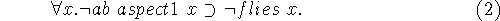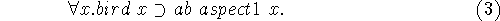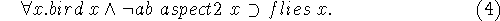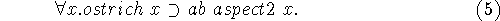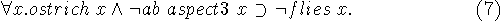Their conjunction is taken as A(ab,flies). This means that what entities satisfy ab and what entities satisfy flies are to be chosen so as to minimize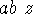. Which objects are birds and ostriches are parameters rather than variables, i.e. what objects are birds is considered given.

We also need an axiom that asserts that the aspects are different. Here is a straightforward version that would be rather long were there more than three aspects.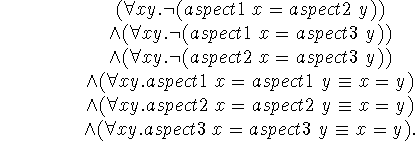We could include this axiom in A(ab,flies), but as we shall see, it won't matter whether we do, because it contains neither ab nor flies. The circumscription formula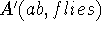is then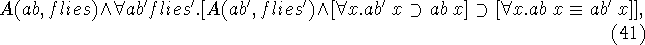which spelled out becomes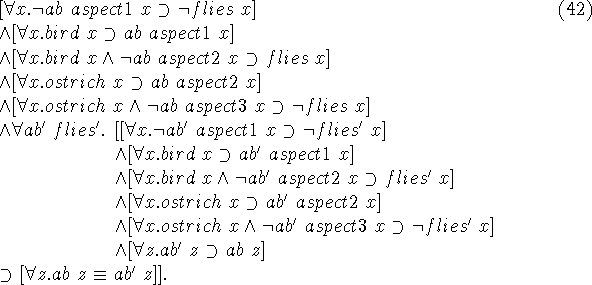A(ab,flies) is guaranteed to be true, because it is part of what is assumed in our common sense database. Therefore (42) reduces to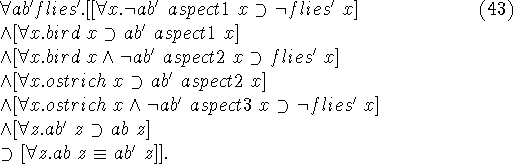Our objective is now to make suitable substitutions for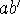and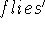so that all the terms preceding the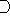in (43) will be true, and the right side will determine ab. The axiom A(ab,flies) will then determine flies, i.e. we will know what the fliers are.is easy, because we need only apply wishful thinking; we want the fliers to be just those birds that aren't ostriches. Therefore, we put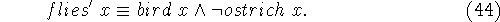isn't really much more difficult, but there is a notational problem. We define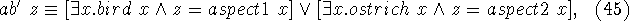which covers the cases we want to be abnormal.

Appendix A contains a complete proof as accepted by Jussi Ketonen's (1984) interactive theorem prover EKL. EKL uses the theory of types and therefore has no problem with the second order logic required by circumscription.Next: SIMPLE ABNORMALITY THEORIES Up: APPLICATIONS OF CIRCUMSCRIPTION Previous: THE BLOCKS WORLD

John McCarthy
Sat Jun 1 13:54:22 PDT 1996